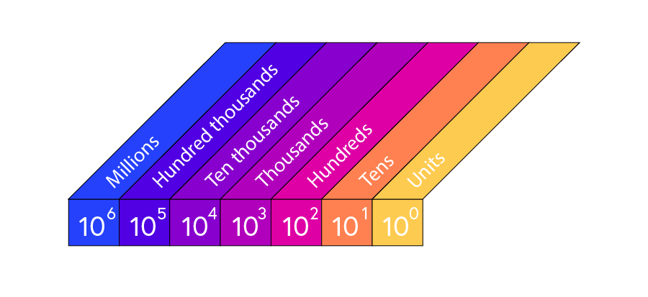1.6

## National STEM Learning Centre

Skip to 0 minutes and 7 secondsPAULA KELLY: So let's have a look at how our place value system actually works.

Skip to 0 minutes and 12 secondsMICHAEL ANDERSON: So in primary school, this will be quite a familiar table. We've got our Units, our Tens, our Hundreds, Thousands, Ten Thousands, et cetera, et cetera, each going up by 10 times the amount, by a power of 10 each time. So if we were to think about the number 18, we would write 1 in the Tens column and 8 in the Units column. And it sounds really obvious to say, but 18 stands for 1 Ten and 8 lots of Units.

Skip to 0 minutes and 40 secondsPAULA KELLY: OK. So could you have-- you could have 9 Units for 19. Could you have 10 units?

Skip to 0 minutes and 47 secondsMICHAEL ANDERSON: Not quite. So our base 10, our place value system, is reliant on number bonds to 10. So if we think of 18, for example, if we added 2 to that, so our Units went from 8 to 9, it will then go back to 0 because 18 add 2 would give us 20. We've now got enough to have 2 lots of Ten and 0 Units.

Skip to 1 minute and 10 secondsPAULA KELLY: OK. So would the same thing work if we had large numbers?

Skip to 1 minute and 14 secondsMICHAEL ANDERSON: Yeah. So it's just a case of making sure that you're consistent, that the Units are here, Tens, Hundreds, Thousands, et cetera. So if we look at, say, 238, that means we've got 2 Hundreds, 3 lots of Ten is 30, and then we've got 8 Units. So 238 is 2 Hundreds, 3 Tens, and 8 Units.

Skip to 1 minute and 37 secondsPAULA KELLY: OK. Even larger still, will it still work?

Skip to 1 minute and 40 secondsMICHAEL ANDERSON: Yeah. So you we can get as big numbers as you like, so long as you can keep on going. So we could have something like 2,317. And it's slightly quirky, this number, because actually we say a 17. But what we mean is 2 Thousands, 3 Hundreds, 1 Ten, and 7 Units.

Skip to 2 minutes and 7 secondsMICHAEL ANDERSON: Well, it's almost exactly the same. We just use different column headings. So here, we've got 10 to the power of 0, and that means our Ones or our Units column. Here, we've got 10 to the power of 1, which is our Tens. And then Hundreds. 10 times 10 is 100. Thousands. Ten Thousands. Hundreds Thousands. And Millions. And we can keep on going and making each column 10 times larger, so a different power of 10.

Skip to 2 minutes and 29 secondsPAULA KELLY: And we're using 10 because our number system is based on our base 10, basically.

Skip to 2 minutes and 34 secondsMICHAEL ANDERSON: Yeah, and again, those number bonds, the 10, tell us when we can move into the next column. So if we had something like, again, 18, that's 1 lot of 10 and 8 Units. And then, once we're counting up, so 19 would still be there. And then we to 20. We've got 2 Tens now and 0 Units. And you can put zeros in as well. So for example, if we had something like 3,105, that means we've got 3 Thousands, 1 Hundred, 0 Tens, and 5 Units.

# How base 10 works

We meet numbers at an early age. It is easy to take numbers for granted. Taking the time to explore exactly what numbers mean, however trivial this may appear, can reap benefits. A sound understanding of place value is vital when getting to grips with rounding, significant figures and standard form.

The standard number base we use is base ten. This means that we group numbers in tens. When counting in base ten we use the digits 0,1,2,3,4,5,6,7,8 and 9. We do not have a separate digit for the number ten. To represent ten as a 1 and a 0 representing one group of ten and no units. Eleven is one ten and one unit: 11. We continue this pattern until we get to twenty which is two groups of ten and no units: 20.

When we get to ten lots of ten we give this the name of one hundred. We now group numbers into hundreds, tens and units e.g. 123 means one hundred, two lots of ten and three units.

In this video, Michael and Paula explain the basics of how numbers work using the base ten place value system we most commonly use today. ‘Placing’ the numbers in the correct column is the key to understanding the ‘place value’. Two sets of column headings are used, one using words and the other, powers of ten. Students should be familiar, and confident, when using either notation.## Problem worksheet

Now complete questions 3 to 6 from this week’s worksheet